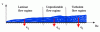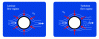I have forgotten

•http://facebook.com/
•https://www.google.com/accounts/o8/id
•https://me.yahoo.com

# Convection

#### Nusselt Flat

Returns the Nusselt number for a fluid going over a flat surface.
 double Nusselt_flat (double Pr, double Re)[inline]#### Nusselt Extpipe

Returns the Nusselt number for a fluid flowing over a pipe.
 double Nusselt_extpipe (double Pr, double Prw, double Re)[inline]#### Nusselt Intpipe

Returns the Nusselt number for a fluid going through a pipe.
 double Nusselt_intpipe (double Pr, double Gr, double Re)[inline]#### Parameters

Module containing parameters used in the convective heat transfer modules.
 double Prandtl (double mu, double a)[inline] double Grashof (double mu, double beta, double dT, double L)[inline] double Reynolds (double mu, double w, double L)[inline]

#### Flow

Calculates the convective heat flow per unit area of a fluid.
 double flow (double lambda, double L, double dT, double Nu)